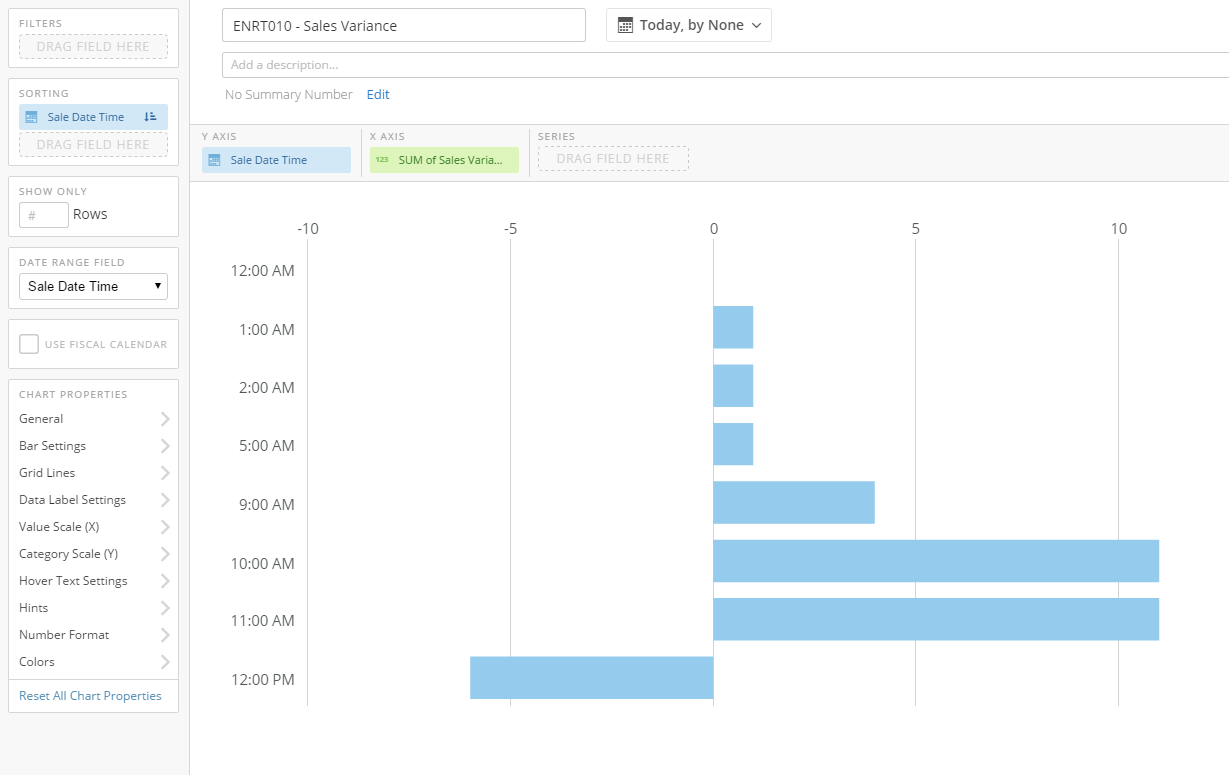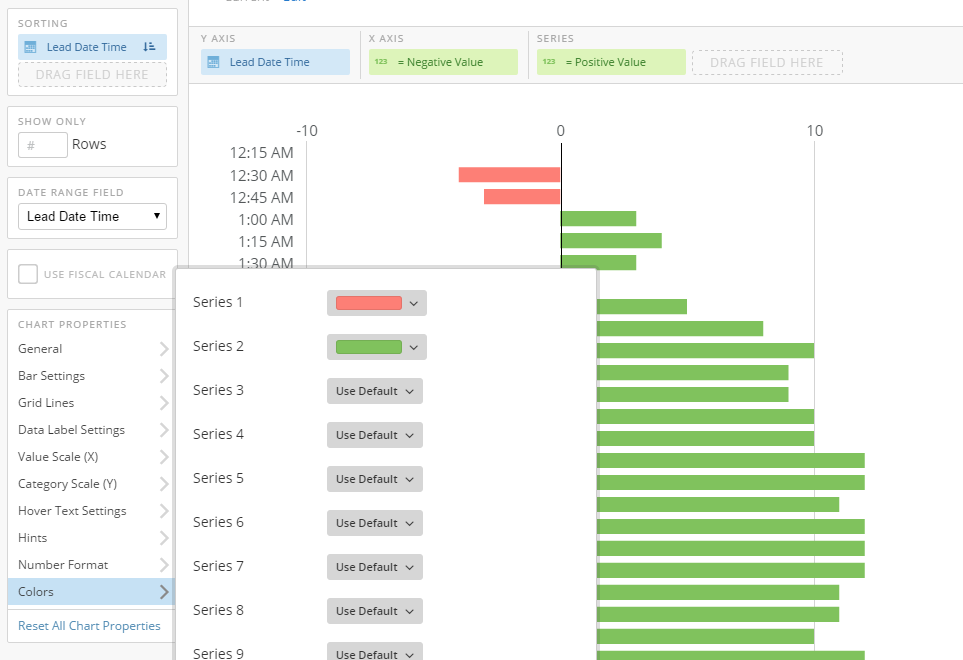# Dynamic colors in bar chart with semi-transactional data

Hello,

I am attempting to create a horizontal bar chart that shows the variance between the sales and sales forecast. A secondary requirement is to be able to filter down by our marketing channels. I have attached the image below of the bar chart I was able to create. The two field used are the Sale Date Time and Sum of Sales Variance. I must be a sum because in the table each row/time combination represents a different marketing channel.

Is it possible to dynamiclly color the bars in the chart green/red for te +/- values? I have seen another post on the community on how to do it but it does not work with hte adding dimension to the data table.• Hi @eizquierdo,

I reached out to you over Buzz and was able to solve the issue with you. If you need to do so, please refer to that Buzz conversation for any infomaton regarding this.

Thank you

Maxwell

Dojo User
**Say "Thanks" by clicking the "heart" in the post that helped you.
**Please mark the post that solves your problem by clicking on "Accept as Solution"
• Thank you for the help.

Solution:

The solution was to set the card as a stacked bar type. Create two beast mode calcuations, one for the positive values and a second for the negative values.

i.e.

Negative Value =  CASE WHEN (SUM(`Sales`) - SUM (`Sales Forecast`)) < 0  THEN SUM(`Sales`) - SUM (`Sales Forecast`) END

Positive Value =  CASE WHEN (SUM(`Sales`) - SUM (`Sales Forecast`)) >= 0  THEN SUM(`Sales`) - SUM (`Sales Forecast`) END

The Date\Time field was added as the Y Axis with the Negative Value in the X Axis and the Positive value as the frist Series. Then in the color options you can set your colors for the two series invovled. FYI - The same soluition may be applied to the single bar chart type however you are not able to hide the legend.•ContributorHi all,

Can anybody help @eizquierdo out?

Thanks!

• Hi @eizquierdo,

I reached out to you over Buzz and was able to solve the issue with you. If you need to do so, please refer to that Buzz conversation for any infomaton regarding this.

Thank you

Maxwell

Dojo User
**Say "Thanks" by clicking the "heart" in the post that helped you.
**Please mark the post that solves your problem by clicking on "Accept as Solution"
• Thank you for the help.

Solution:

The solution was to set the card as a stacked bar type. Create two beast mode calcuations, one for the positive values and a second for the negative values.

i.e.

Negative Value =  CASE WHEN (SUM(`Sales`) - SUM (`Sales Forecast`)) < 0  THEN SUM(`Sales`) - SUM (`Sales Forecast`) END

Positive Value =  CASE WHEN (SUM(`Sales`) - SUM (`Sales Forecast`)) >= 0  THEN SUM(`Sales`) - SUM (`Sales Forecast`) END

The Date\Time field was added as the Y Axis with the Negative Value in the X Axis and the Positive value as the frist Series. Then in the color options you can set your colors for the two series invovled. FYI - The same soluition may be applied to the single bar chart type however you are not able to hide the legend.This discussion has been closed.